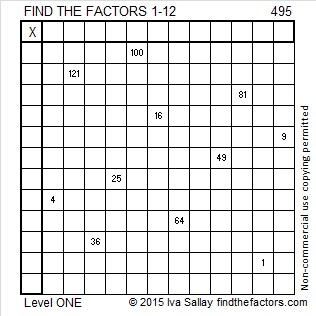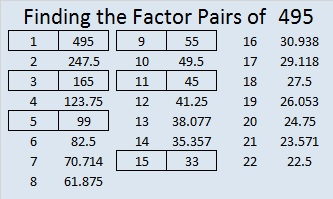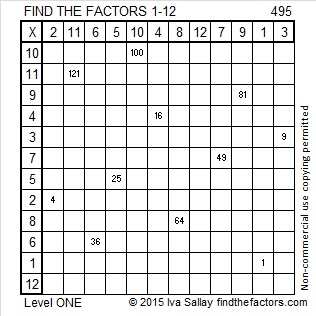# 495 and Level 1

495 has an almost magical property and is known as the Kaprekar constant for 3 digit numbers. What does that mean?

Take any 3 digit number that has at least 2 different digits. Write the digits from greatest to least to create a new 3-digit number. From that number subtract the same digits written in reverse order. Repeat this process, and you will get the number 495 in no more than seven iterations. This graphic shows this process applied to the number 101.The following puzzle can easily transform into a multiplication table if you first find all the square factors of the given clues. Go ahead give it a try!Print the puzzles or type the solution on this excel file: 12 Factors 2015-05-18

—————————————————————————————————

• 495 is a composite number.
• Prime factorization: 495 = 3 x 3 x 5 x 11, which can be written 495 = (3^2) x 5 x 11
• The exponents in the prime factorization are 2, 1, and 1. Adding one to each and multiplying we get (2 + 1)(1 + 1)(1 + 1) = 3 x 2 x 2 = 12. Therefore 495 has exactly 12 factors.
• Factors of 495: 1, 3, 5, 9, 11, 15, 33, 45, 55, 99, 165, 495
• Factor pairs: 495 = 1 x 495, 3 x 165, 5 x 99, 9 x 55, 11 x 45, or 15 x 33
• Taking the factor pair with the largest square number factor, we get √495 = (√9)(√55) = 3√55 ≈ 22.248595—————————————————————————————————